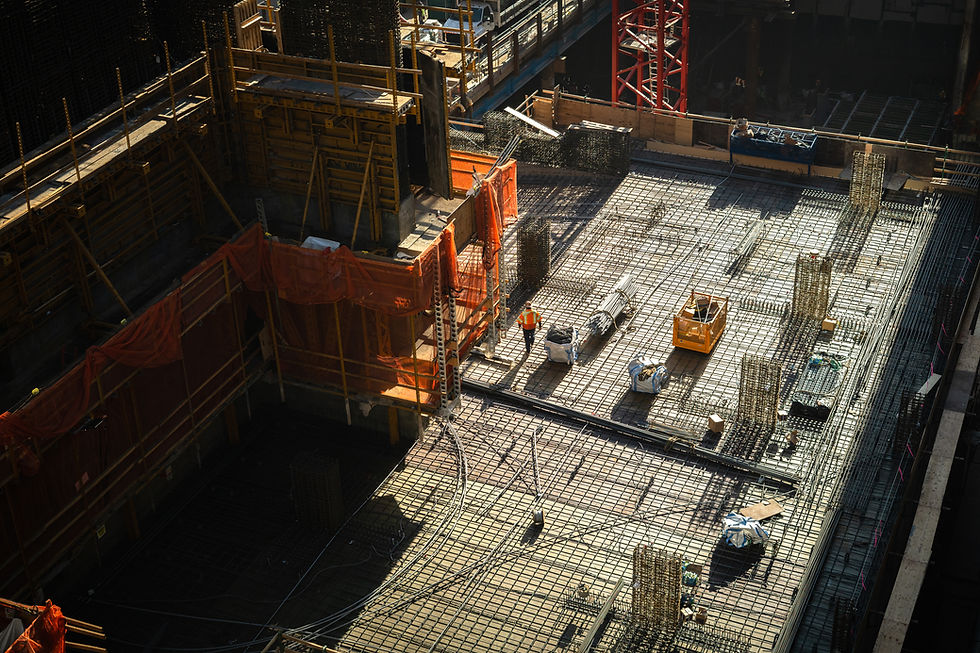top of page###### Complex Payback Period

Accounting (Year 12) - Capital Expenditure

Christian Bien

# How Do We Calculate Payback Period Where the Cash Flows are Different Each Year?

The previous page show how to calculate Payback Period where Cash Flow is consistent each year.

For an asset where cash flows are different each year, the payback period are calculated by deducting the cash inflow from the initial cash outflow.

# Worked Example: Complex Payback Period Calculation

Below is an example of how to calculate a Payback Period where cash flows are not consistent each year. Johns Plumbing Suppliers produces pipes for industrial applications. He is considering investing \$100,000 into a new pipe manufacturing equipment.

The expected cash flows (listed above) will generate the following cash inflows for his business:

• Year 1: \$20,000

• Year 2: \$30,000

• Year 3: \$40,000

• Year 4: \$40,000

• Year 5: \$30,000

There is no salvage value at the end of its useful life at Year 5.

Johns Plumbing Suppliers' acceptable payback period is less than 4 years.

# Step 1: Calculate the Accumulative Cash Flows

The first thing we do is to determine the accumulative cash flows - in other words, we stack each year's cash inflow as we go.

Therefore:

• Year 1 = \$20,000

• Year 2 = Year 1 Cash Inflow + Year 2 Cash Inflow = \$20,000 + \$30,000 = \$50,000

• Year 3 = Year 1 Cash Inflow + Year 2 Cash Inflow + Year 3 Cash Inflow = \$20,000 + \$30,000 + \$40,000 = \$90,000

And so on... See the above image for the calculated accumulative cash flow. We do this to determine two things: 1. If there is a payback period - If the accumulated cash flow is less than the initial investment, then there is no payback period, as the cash generated does not exceed the initial investment. 2. The low-high point (see next step).

# Step 2: Determine the Low-High point in Cash Flow

The next step is we have to determine the high point, the point where the accumulative cash flows exceeds the initial investment. The initial investment of \$100,000 is exceeded at Year 4, where accumulative cash flow is \$130,000. This is the high point.

The subsequent low point, is the period where the accumulative cash flow is just below the initial investment. This is the point at Year 3 at \$90,000. Therefore, we know that the payback period occurs between Year 3 and Year 4.

# Step 3: Calculate the Partial Year

Since we know the Low-High point, we know the payback period is between Year 3 and Year 4. Now its time to calculate the partial year of which the payback period is achieved. We know at Year 3 we have accumulated cash inflows of \$90,000.

Therefore, the remaining amount is: = Initial Investment - Acc Cash Inflow at Low Point = \$100,000 - \$90,000 = \$10,000 As Year 4 the cash inflow is \$40,000, this exceeds the remaining \$10,000 and hence, we must do a proportion of this amount. I.e \$10,000/\$40,000 = 0.25 years. Hence, at 0.25 years after year 3, the payback period is achieved.

# Step 4: Put it Together to Calculate the Payback Period

We know that it takes 0.25 years to achieve the payback period between years 3 and 4. We must convert this into months. To do this, simply take the years and multiply it by the amount of months in a year (12).

Hence, 0.25 years * 12 months = 3 months. Afterwards, put it together with the low point year, you get 3 years and 3 months.

This investment should be accepted as it is less than the acceptable period of 4 years stated in the question. Hint: There is a perfect month calculation with no remainders. If there is a decimal, always round up.

For example, if it was 2.3 months, always round up. 2.3 months would become 2 months. If you put 2 months, technically, the payback period hasn't been achieved as there would still be a remaining 0.3 months on the investment.

bottom of page